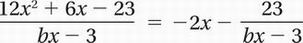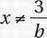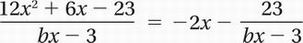# SAT Math Multiple Choice Question 656: Answer and Explanation

### Test Information

Question: 656

11. The equationis true for all values of, where b is a constant. What is the value of b?

• A. -12
• B. -6
• C. 4
• D. 6

Explanation:

B

The fact that the denominators are equal in both fractions suggests that multiplying both sides will simplifyMultiply by

bx - 3: 12x2 + 6x - 23 = -2x(bx - 3) - 23

Distribute:

12x2 + 6x - 23 = -2bx2 + 6x - 23

Subtract 6x - 23:

12x2 = -2bx2

Divide by x2:

12 = -2b